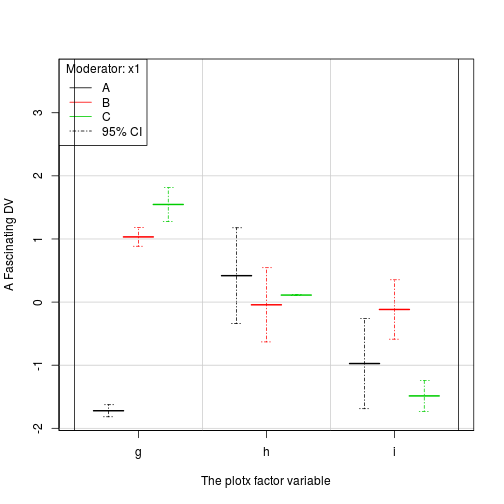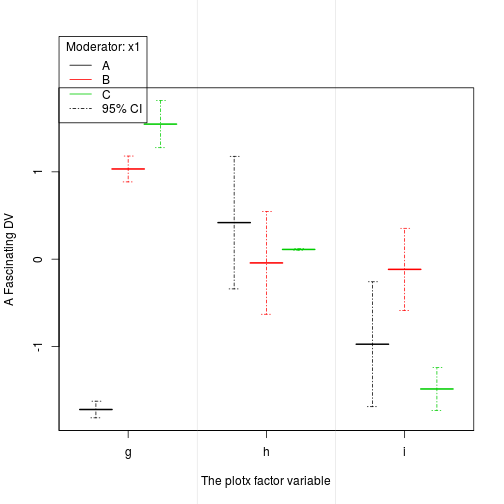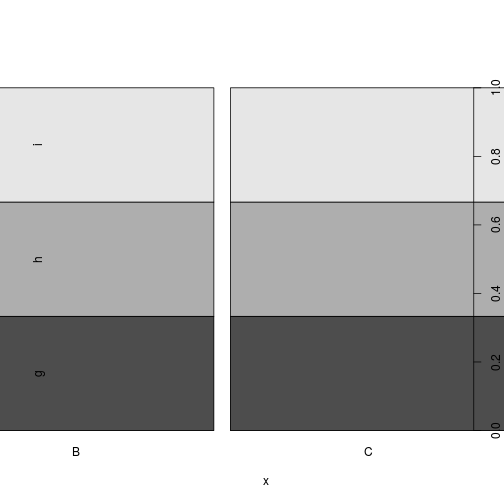``````## plot-regression-factors-1.R
## Paul Johnson
## 2013-03-30

## Students suggest a plot for interactions of factor variables
## to replace plotSlopes when plotx is categorical. There will
## be 2 factor predictors in a regression, and we want to
## display them in a style that is familiar to psychologists.

## I think this just ends up being an idiosyncratic version of
## a dotchart, but its mine.

## First, make up some data:
x1 <- factor(c("A","B","C","A","B","C","A","B","C"))
x2 <- factor(c("g","g","g","h","h","h","i","i","i"))
y <- rnorm(9)

err <- runif(9)
datpr <- data.frame(x1, x2, fit = y, lwr = y - err, upr = y + err)
rm(x1, x2, err, y)

SAVEME <- FALSE

## sp: space between factor displays
sp <- 0.3
x1w <- (1 - sp) / length(levels(datpr\$x1))

x1l <- length(levels(datpr\$x1))
x2l <- length(levels(datpr\$x2))

if (SAVEME) pdf(file="plot-factor-proto-1.pdf", height = 6, width = 6, paper = "special", onefile=F)
plot(x = 1:2, y = 1:2,  xlim = c(0.5, 3.5), ylim = c(min(datpr\$lwr), 2 * max(datpr\$upr)), type = "n", xaxt = "n", bty = "L", xlab = "The plotx factor variable", ylab = "A Fascinating DV")

axis(1, at = 1:length(levels(datpr\$x2)), labels = levels(datpr\$x2))

mapply(FUN = function(x1, x2, fit) {
lines(x = c(x2 - 0.5 + 0.5 * sp + x1w * (x1-1), x2 - 0.5 + 0.5 * sp + x1w * x1),
y = rep(fit, 2), col = x1, lwd = 2)
}, x1 = as.numeric(datpr\$x1), x2 = as.numeric(datpr\$x2), fit = datpr\$fit)
``````
``````## []
## NULL
##
## []
## NULL
##
## []
## NULL
##
## []
## NULL
##
## []
## NULL
##
## []
## NULL
##
## []
## NULL
##
## []
## NULL
##
## []
## NULL
``````
``````mapply(FUN = function(x1, x2, lwr, upr) {
arrows(x0 = x2 - 0.5 + 0.5 * sp + x1w * (x1 - 1/2),
x1 = x2 - 0.5 + 0.5 * sp + x1w * (x1 - 1/2),
y0 = lwr, y1 = upr, code = 3, angle = 90, length = 0.07, lty = 4, col = x1)
}, x1 = as.numeric(datpr\$x1), x2 = as.numeric(datpr\$x2), lwr = datpr\$lwr, upr = datpr\$upr)
``````
``````## []
## NULL
##
## []
## NULL
##
## []
## NULL
##
## []
## NULL
##
## []
## NULL
##
## []
## NULL
##
## []
## NULL
##
## []
## NULL
##
## []
## NULL
``````
``````legend("topleft", legend = c(levels(datpr\$x1), "95% CI"), lty = c(1,1,1,4), col = c(1:3,1), title = "Moderator: x1", bg = "white")

abline(v = seq(1.5, 1.5 + (x2l - 2), by = 1), lwd = 1, col = gray(.8))

## What could be better?

## Would we like some horizontal lines?
abline(h = pretty(datpr\$fit, 4), col = gray(.8))

## Do we want the box to go on all 4 sides?  Somehow, I like the L shaped
## box, but this certainly looks more standard
box()

if (SAVEME) dev.off()

## Are we bothered that R inserts some space on the left and right
## edges of the graph?  I intended the x axis to allow equal space to the
## levels of plotx, but R automatically inserts some space for me 4%,
## and I don't know how to get rid of it (yet). To see where the limits
## would be to make this symmetric

abline(v = c(0.5, x2l + 0.5))
````````````## Here is the fix for that. Run this command
par(xaxs = "i")
## and then run the plot sequence again

## Are you bothered that I inserted a big block of empty space
## at the top of the graph so that I had room for a legend?

## Would you prefer this style instead?
if (SAVEME) pdf(file="plot-factor-proto-2.pdf", height = 6, width = 6, paper = "special", onefile=F)
par(xpd = TRUE, mar = par("mar") + c(0,0,2,0), xaxs = "i")
plot(x = 1:2, y = 1:2,  xlim = c(0.5, 3.5), ylim = c(min(datpr\$lwr),  max(datpr\$upr)), type = "n", xaxt = "n", bty = "L", xlab = "The plotx factor variable", ylab = "A Fascinating DV")

axis(1, at = 1:length(levels(datpr\$x2)), labels = levels(datpr\$x2))

mapply(FUN = function(x1, x2, fit) {
lines(x = c(x2 - 0.5 + 0.5 * sp + x1w * (x1-1), x2 - 0.5 + 0.5 * sp + x1w * x1),
y = rep(fit, 2), col = x1, lwd = 2)
}, x1 = as.numeric(datpr\$x1), x2 = as.numeric(datpr\$x2), fit = datpr\$fit)
``````
``````## []
## NULL
##
## []
## NULL
##
## []
## NULL
##
## []
## NULL
##
## []
## NULL
##
## []
## NULL
##
## []
## NULL
##
## []
## NULL
##
## []
## NULL
``````
``````mapply(FUN = function(x1, x2, lwr, upr) {
arrows(x0 = x2 - 0.5 + 0.5 * sp + x1w * (x1 - 1/2),
x1 = x2 - 0.5 + 0.5 * sp + x1w * (x1 - 1/2),
y0 = lwr, y1 = upr, code = 3, angle = 90, length = 0.07, lty = 4, col = x1)
}, x1 = as.numeric(datpr\$x1), x2 = as.numeric(datpr\$x2), lwr = datpr\$lwr, upr = datpr\$upr)
``````
``````## []
## NULL
##
## []
## NULL
##
## []
## NULL
##
## []
## NULL
##
## []
## NULL
##
## []
## NULL
##
## []
## NULL
##
## []
## NULL
##
## []
## NULL
``````
``````legend(par("usr"), 1.3* par("usr"), legend = c(levels(datpr\$x1), "95% CI"), lty = c(1,1,1,4), col = c(1:3,1), title = "Moderator: x1", bg = "white")

abline(v = seq(1.5, 1.5 + (x2l - 2), by = 1), lwd = 1, col = gray(.9))

## If you like that, please note that you just really cannot
## have a box. This is bad:
if (SAVEME) dev.off()

box()
````````````## Along the way, I noticed a weirdness in the plot method for
## factors. type="n" is not accepted.
plot(x = datpr\$x1, y = datpr\$x2,  xlim = c(0.5, 3.75), ylim = range(datpr\$y), type = "n")
``````
``````## Warning: no non-missing arguments to min; returning Inf
## Warning: no non-missing arguments to max; returning -Inf
## Warning: x axis is on a cumulative probability scale, 'xlim' must be in [0,1]
## Warning: y axis is on a cumulative probability scale, 'ylim' must be in [0,1]
## Warning: graphical parameter "type" is obsolete
````````````## Wow. Try with simpler example:
## plot(x = factor(c("A","B")), y = factor(c("x","y")), type= "n")
## Warning message:
## In rect(xleft, ybottom, xright, ytop, col = col, ...) :
##   graphical parameter "type" is obsolete

## begin at (i-1) + 0.25, (i-1) + (1/#j)
``````